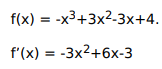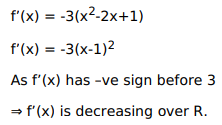# Solve this following

Question:

Mark $(\sqrt{)}$ against the correct answer in the following:

The function $f(x)=4-3 x+3 x^{2}-x^{3}$ is

A. decreasing on $\mathrm{R}$

B. increasing on $\mathrm{R}$

C. strictly decreasing on $R$

D. strictly increasing on $R$

Solution: